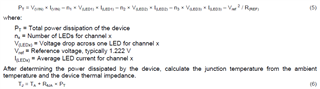If you have a related question, please click the "Ask a related question" button in the top right corner. The newly created question will be automatically linked to this question.

# TPS92630-Q1: Reg. Power Dissipation Calc.

Part Number: TPS92630-Q1

Hi,

As per the Datasheet of TPS92630, the Power dissipation of the IC is as given below. Pls help me understanding the I(VIN). should I consider the quiescent current to calculate the Pd??

If I consider Iq, then i get wrong value.

My requirement is to calculate the Pd for Vin = 12V, I(LED) = 50mA and all 8 channels are loaded with LEDs (3/channel), Vf(LED) = 2.5 V/LED.Pls revert at the earliest.

• My requirement is to calculate the Pd for Vin = 12V, I(LED) = 50mA and all 8 channels are loaded with LEDs (3/channel), Vf(LED) = 2.5 V/LED

We only have 3 channels, and the device consume little power by itself.

•  Sorry, i will be connecting 3 LEDs/channel. What is meant by I(VIN) in the Power dissipation formula given in the Datasheet. if I consider the quiescent current, the result is going in negative.

Pd = (12 * 0.00085) - (3* 7.5 * 0.05) - (1.222^2/7400) = The result is negative. Is my way of calculating the Pd correct?? What should be the I(VIN)??

• I(Vin) should include the current flow through the LED, so your calculation way is wrong.